# 基于极限环的四旋翼空间圆形编队控制Finite-Time Spatial Circle Formation Control of Quadrotor Systems Based on Limit Cycle

DOI: 10.12677/DSC.2020.93013, PDF, HTML, XML, 下载: 98  浏览: 574  国家自然科学基金支持

Abstract: In this paper, a quadrotor formation system with limited sensing ability is capable of moving unanimously to form the desired circular formation only using the position information of the target center of the circle and the neighboring quadrotors. By employing a method of decoupling control based on limit-cycle, the spatial circle formation is decoupled into three sub-problems: height control, circular convergence, and hovering together with the spacing distribution. Then a distributed controller composed of height control, circular convergence control, and spacing distribution control is designed to drive the quadrotors to form a circle in the space. This controller has the collision-avoidance among the quadrotors under consideration as well. It can constitute the desired circular distribution of relative position at the designated height and hold the desired formation when the quadrotors fly around the target. Lastly, the effectiveness of the control law is verified by simulations and experiments.

1. 引言

2. 四旋翼建模

2.1. 四旋翼数学模型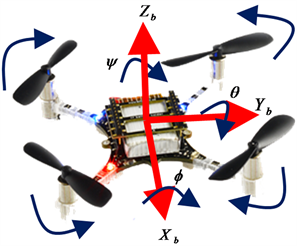Figure 1. Schematic diagram of type X quadrotorTable 1. Parameters and their description

$\left\{\begin{array}{l}{\tau }_{x}=\sqrt{2}{C}_{T}l\left(-{\omega }_{1}^{2}+{\omega }_{2}^{2}+{\omega }_{3}^{2}-{\omega }_{4}^{2}\right)/2\hfill \\ {\tau }_{y}=\sqrt{2}{C}_{T}l\left({\omega }_{1}^{2}-{\omega }_{2}^{2}+{\omega }_{3}^{2}-{\omega }_{4}^{2}\right)/2\hfill \\ {\tau }_{z}={C}_{D}\left(+{\omega }_{1}^{2}+{\omega }_{2}^{2}-{\omega }_{3}^{2}-{\omega }_{4}^{2}\right)\hfill \end{array}$ (1)

$\left\{\begin{array}{l}{\stackrel{¨}{x}}_{\text{e}}={F}_{\text{sum}}\left(\mathrm{cos}\phi \mathrm{sin}\theta \mathrm{cos}\psi +\mathrm{sin}\phi \mathrm{sin}\psi \right)/m\hfill \\ {\stackrel{¨}{y}}_{\text{e}}={F}_{\text{sum}}\left(\mathrm{cos}\phi \mathrm{sin}\theta \mathrm{sin}\psi +\mathrm{sin}\phi \mathrm{cos}\psi \right)/m\hfill \\ {\stackrel{¨}{z}}_{\text{e}}={F}_{\text{sum}}\mathrm{cos}\phi \mathrm{cos}\theta /m-g\hfill \\ \stackrel{¨}{\phi }=\left({I}_{y}-{I}_{z}\right)\stackrel{˙}{\theta }\stackrel{˙}{\psi }/{I}_{x}+{\tau }_{x}/{I}_{x}\hfill \\ \stackrel{¨}{\theta }=\left({I}_{z}-{I}_{x}\right)\stackrel{˙}{\phi }\stackrel{˙}{\psi }/{I}_{y}+{\tau }_{y}/{I}_{y}\hfill \\ \stackrel{¨}{\psi }=\left({I}_{x}-{I}_{y}\right)\stackrel{˙}{\phi }\stackrel{˙}{\theta }/{I}_{z}+{\tau }_{z}/{I}_{z}\hfill \end{array}$ (2)

$\left[\begin{array}{c}{\stackrel{¨}{x}}_{\text{b}}\\ {\stackrel{¨}{y}}_{\text{b}}\end{array}\right]=\left[\begin{array}{cc}\mathrm{cos}\psi & -\mathrm{sin}\psi \\ \mathrm{sin}\psi & \mathrm{cos}\psi \end{array}\right]\left[\begin{array}{c}{\stackrel{¨}{x}}_{\text{e}}\\ {\stackrel{¨}{y}}_{\text{e}}\end{array}\right]$ (3)

$\left\{\begin{array}{l}\phi =\mathrm{arctan}\left(-\left({\stackrel{¨}{x}}_{\text{e}}\mathrm{cos}\psi +{\stackrel{¨}{y}}_{\text{e}}\mathrm{sin}\psi \right)/g\right)\\ \theta =\mathrm{arctan}\left(\left(-{\stackrel{¨}{x}}_{\text{e}}\mathrm{sin}\psi +{\stackrel{¨}{y}}_{\text{e}}\mathrm{cos}\psi \right)\mathrm{cos}\phi /g\right)\end{array}$ (4)

2.2. 系统感知拓扑结构及队形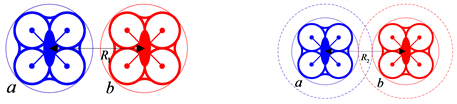Figure 2. (a) Minimum safety distance; (b) Collision avoidance distance

${\stackrel{¯}{p}}_{i}={p}_{i}-{p}_{0}={\left[\begin{array}{ccc}{\stackrel{¯}{x}}_{i}& {\stackrel{¯}{y}}_{i}& {\stackrel{¯}{z}}_{i}\end{array}\right]}^{\text{T}},\text{}i\in {I}_{n}$ (5)

${\stackrel{¯}{p}}_{i}=\left[\begin{array}{c}{\rho }_{i}\mathrm{cos}{\alpha }_{i}\\ \begin{array}{c}{\rho }_{i}\mathrm{sin}{\alpha }_{i}\\ {\stackrel{¯}{z}}_{i}\end{array}\end{array}\right],\text{}i\in {I}_{n}$ (6)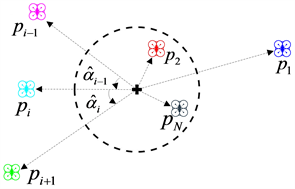Figure 3. Top view of the quadrotor space system number

$i+1=\left\{\begin{array}{l}i+1,\text{}i=1,2,\cdots ,N-1\hfill \\ 1,\text{}i=N\hfill \end{array}$

$i-1=\left\{\begin{array}{l}i-1,\text{}i=2,3,\cdots ,N\hfill \\ N,\text{}i=1\hfill \end{array}$ (7)

$\left\{\begin{array}{l}{\stackrel{^}{p}}_{i}={p}_{i+1}-{p}_{i}\hfill \\ {\stackrel{^}{p}}_{i-1}={p}_{i}-{p}_{i-1}\hfill \end{array}\text{,}i\in {I}_{n}$ (8)

$d={\left[{d}_{1},{d}_{2},\cdots ,{d}_{N}\right]}^{\text{T}}\text{,}{\sum }_{i=1}^{N}{d}_{i}=2\pi$ (9)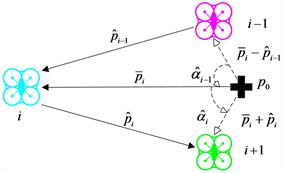Figure 4. Top view of topological structure information perceptible by each quadrotor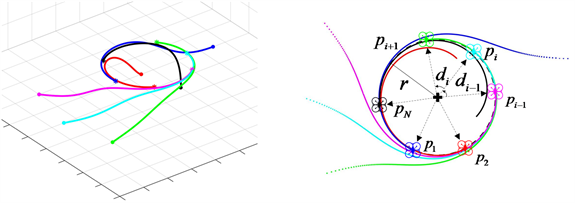Figure 5. (a) Space formation trajectory; (b) Top view of quadrotor space trajectory and expected formation description

${\stackrel{˙}{\stackrel{¯}{p}}}_{i}=\left[\begin{array}{c}{\stackrel{˙}{\stackrel{¯}{x}}}_{\text{e}i}\\ {\stackrel{˙}{\stackrel{¯}{y}}}_{\text{e}i}\\ {\stackrel{˙}{\stackrel{¯}{z}}}_{\text{e}i}\end{array}\right]=\left[\begin{array}{c}{u}_{xi}\\ {u}_{yi}\\ {u}_{zi}\end{array}\right],\text{}i\in {I}_{n}$ (10)

$\left\{\begin{array}{l}\begin{array}{l}{z}_{i}^{*}=h\text{}\left(高度控制\right)\\ {\rho }_{i}^{*}=r\text{}\left(目标盘旋\right)\end{array}\hfill \\ {\stackrel{^}{\alpha }}_{i}^{*}={d}_{i}\text{}\left(间距调整\right)\hfill \end{array},\text{\hspace{0.17em}}\text{\hspace{0.17em}}i\in {I}_{n}$ (11)

3. 控制器设计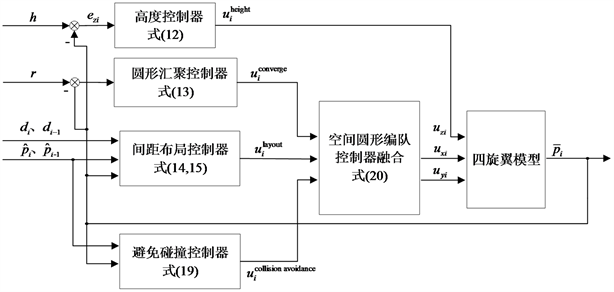Figure 6. Block diagram of the quadrotors space circular formation controller

3.1. 高度控制

${u}_{i}^{\text{height}}={K}_{\text{P}}\left({e}_{zi}+\frac{1}{{K}_{\text{I}}}\int {e}_{zi}\text{d}t+\frac{{K}_{\text{D}}\text{d}{e}_{zi}}{\text{d}t}\right),\text{}i\in {I}_{n}$ (12)

3.2. 圆形汇聚控制

${u}_{i}^{\text{converge}}=\lambda \left[\begin{array}{cc}\gamma \left({r}^{2}-{‖{\stackrel{¯}{p}}_{i}‖}^{2}\right)& -\text{1}\\ \text{1}& \gamma \left({r}^{2}-{‖{\stackrel{¯}{p}}_{i}‖}^{2}\right)\end{array}\right]\left[\begin{array}{c}{\stackrel{¯}{x}}_{\text{e}i}\\ {\stackrel{¯}{y}}_{\text{e}i}\end{array}\right],\text{}i\in {I}_{n}$ (13)

3.3. 间距布局控制

${u}_{i}^{\text{distribution}}={\left(\frac{{d}_{i-1}}{{d}_{i}+{d}_{i-1}}{\stackrel{^}{\alpha }}_{i}-\frac{{d}_{i}}{{d}_{i}+{d}_{i-1}}{\stackrel{^}{\alpha }}_{i-1}\right)}^{\alpha },\text{}i\in {I}_{n}$ (14)

${u}_{i}^{\text{layout}}={c}_{1}+\frac{{c}_{2}}{2\pi }{u}_{i}^{\text{distribution}},\text{}i\in {I}_{n}$ (15)

3.4. 避免碰撞控制

${P}_{ij}\left({p}_{i},{p}_{j}\right)={\left(\mathrm{min}\left\{0,\frac{{‖{p}_{i}-{p}_{j}‖}^{2}-{R}_{2}^{2}}{{‖{p}_{i}-{p}_{j}‖}^{2}-{R}_{1}^{2}}\right\}\right)}^{2}\text{},\text{}i,j\in {I}_{n}$ (16)

$\frac{\partial {P}_{ij}}{\partial {p}_{i}}=\left\{\begin{array}{l}0\text{,}‖{p}_{i}-{p}_{j}‖\ge {R}_{2}\hfill \\ \frac{4\left({R}_{2}^{2}-{R}_{1}^{2}\right)\left({‖{p}_{i}-{p}_{j}‖}^{2}-{R}_{2}^{2}\right)\left({p}_{i}-{p}_{j}\right)}{{\left({‖{p}_{i}-{p}_{j}‖}^{2}-{R}_{1}^{2}\right)}^{3}},\text{}{R}_{1}<‖{p}_{i}-{p}_{j}‖<{R}_{2}\hfill \end{array}$ (17)

${P}_{i}={P}_{i,i-1}+{P}_{i,i+1},\text{}i\in {I}_{n}$ (18)

${u}_{i}^{\text{collisionavoidance}}=-\left(\frac{\partial {P}_{i,i-1}}{\partial {p}_{i}}+\frac{\partial {P}_{i,i+1}}{\partial {p}_{i}}\right),\text{}i\in {I}_{n}$ (19)

3.5. 空间圆形编队控制器融合

${\stackrel{˙}{\stackrel{¯}{p}}}_{i}=\left[\begin{array}{l}{u}_{xi}\hfill \\ {u}_{yi}\hfill \\ {u}_{zi}\hfill \end{array}\right]=\left[\begin{array}{c}{u}_{i}^{\text{converge}}×{u}_{i}^{\text{layout}}+\beta {u}_{i}^{\text{collisionavoidance}}{}_{\left(2×1\right)}\\ {u}_{i}^{\text{height}}\end{array}\right],\text{}i\in {I}_{n}$ (20)

$\left[\begin{array}{c}{\stackrel{¨}{x}}_{\text{e}}\\ {\stackrel{¨}{y}}_{\text{e}}\end{array}\right]=\left[\begin{array}{c}{\stackrel{˙}{u}}_{xi}\\ {\stackrel{˙}{u}}_{yi}\end{array}\right],\text{}i\in {I}_{n}$ (21)

4. 仿真与实验Table 2. Space circular formation parameters

4.1. 仿真结果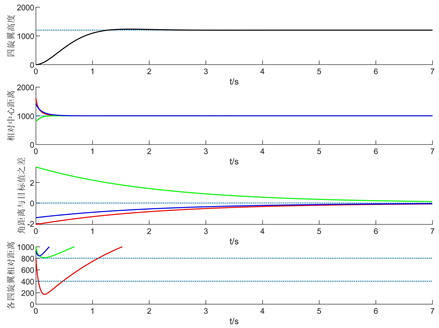Figure 7. For parameter $\alpha =1,\beta =0$, the simulation diagram is formed by the asymptotic distribution of circular relative positions without avoiding collision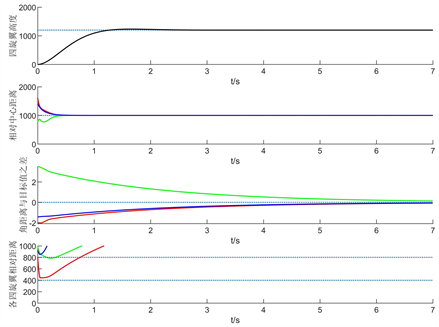Figure 8. For parameter $\alpha =1,\beta =200$, the simulation diagram is formed by the asymptotic distribution of circular relative positions and avoiding collision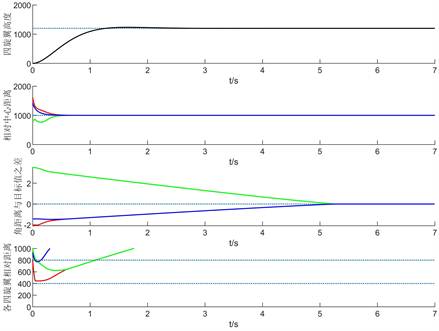Figure 9. For parameter $\alpha =0.1,\beta =200$, the simulation diagram is formed by circular relative positions and avoiding collision in limited time

4.2. 实验验证Figure 10. Actual flight rendering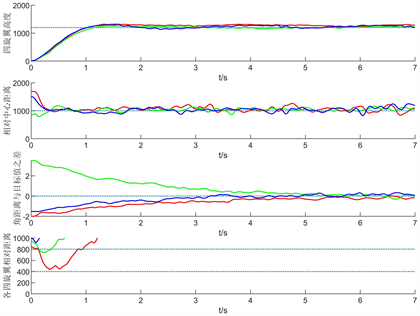Figure 11. The actual flight data record graph formed by the asymptotic distribution of circular relative position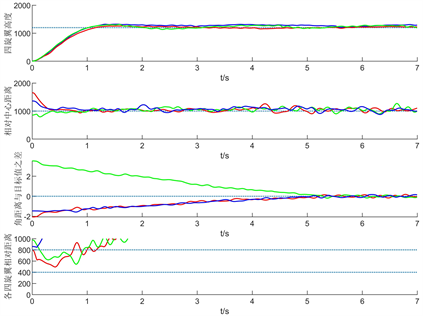Figure 12. The actual flight data record graph formed in a finite time by the circular relative position distribution

5. 总结与展望

NOTES

*通讯作者。

  Alonsomora, J., Naegeli, T., Siegwart, R. and Beardsley, P. (2015) Collision Avoidance for Aerial Vehicles in Multi-Agent Scenarios. Autonomous Robots, 39, 101-121. https://doi.org/10.1007/s10514-015-9429-0  Monteiro, S.N. and Bicho, E. (2008) Robot Formations: Robots Allocation and Leader-Follower Pairs. 2008 IEEE International Conference on Robotics and Automation, Pasadena, CA, 19-23 May 2008, 3769-3775. https://doi.org/10.1109/ROBOT.2008.4543789  Hanada, Y., Lee, G. and Chong, N.Y. (2007) Adaptive Flocking of a Swarm of Robots Based on Local Interactions. 2007 IEEE Swarm Intelligence Symposium, Honolulu, HI, 1-5 April 2007, 340-347. https://doi.org/10.1109/SIS.2007.367957  Sorensen, N. and Ren, W. (2007) A Unified Formation Control Scheme with a Single or Multiple Leaders. 2007 American Control Conference, New York, 9-13 July 2007, 5412-5418. https://doi.org/10.1109/ACC.2007.4283094  Lewis, M.A. and Tan, K.H. (1997) High Precision Formation Control of Mobile Robots Using Virtual Structures. Autonomous Robots, 4, 387-403. https://doi.org/10.1023/A:1008814708459  Low, C.B. and Ng, Q.S. (2011) A Flexible Virtual Structure Formation Keeping Control for Fixed-Wing UAVs. 2011 9th IEEE International Conference on Control and Automation, Santiago, Chile, 19-21 December 2011, 621-626. https://doi.org/10.1109/ICCA.2011.6137876  Seo, J., Ahn, C. and Kim, Y. (2009) Controller Design for UAV Formation Flight Using Consensus Based Decentralized Approach. AIAA Infotech@Aerospace Conference, Seattle, WA, 6-9 April 2009, 1826. https://doi.org/10.2514/6.2009-1826  Lechevin, N., Rabbath, C.A. and Earon, E. (2007) Towards Decentralized Fault Detection in UAV Formations. 2007 American Control Conference, New York, 9-13 July 2007, 5759-5764. https://doi.org/10.1109/ACC.2007.4282194  Hu, Z. and Yang, J. (2019) Distributed Efficiency-Based Circular Formation for the Unmanned Air Vehicles Detection System. Proceedings of the Institution of Mechanical Engineers Part G Journal of Aerospace Engineering, 233, 3223-3234. https://doi.org/10.1177/0954410018793764  Suzuki, I. and Yamashita, M. (2006) Distributed Anonymous Mobile Robots: Formation of Geometric Patterns. SIAM Journal on Computing, 36, 279-280. https://doi.org/10.1137/050631562  Defago, X. and Souissi, S. (2008) Non-Uniform Circle Formation Algorithm for Oblivious Mobile Robots with Convergence toward Uniformity. Theoretical Computer Science, 396, 97-112. https://doi.org/10.1016/j.tcs.2008.01.050  Flocchini, P., Prencipe, G. and Santoro, N. (2008) Self-Deployment of Mobile Sensors on a Ring. Theoretical Computer Science, 402, 67-80. https://doi.org/10.1016/j.tcs.2008.03.006  Wang, C., Xie, G. and Cao, M. (2013) Forming Circle Formations of Anonymous Mobile Agents with Order Preservation. IEEE Transactions on Automatic Control, 58, 3248-3254. https://doi.org/10.1109/TAC.2013.2263653  Wang, C., Xie, G. and Cao, M. (2014) Controlling Anonymous Mobile Agents with Unidirectional Locomotion to Form Formations on a Circle. Automatica, 50, 1100-1108. https://doi.org/10.1016/j.automatica.2014.02.036  Wang, C. and Xie, G. (2017) Limit-Cycle-Based Decoupled Design of Circle Formation Control with Collision Avoidance for Anonymous Agents in a Plane. IEEE Transactions on Automatic Control, 62, 6560-6567. https://doi.org/10.1109/TAC.2017.2712758  Bhat, S.P. and Bernstein, D.S. (2000) Finite-Time Stability of Continuous Autonomous Systems. SIAM Journal on Control and Optimization, 38, 751-766. https://doi.org/10.1137/S0363012997321358  Luis, C. and Ny, J.L. (2016) Design of a Trajectory Tracking Controller for a Nano Quadcopter. arXiv Preprint arXiv:1608.05786.  Stipanovic, D.M., Hokayem, P., Spong, M.W. and Siljak, D.D. (2007) Cooperative Avoidance Control for Multiagent Systems. Journal of Dynamic Systems Measurement and Control-Transactions of the ASME, 129, 699-707. https://doi.org/10.1115/1.2764510